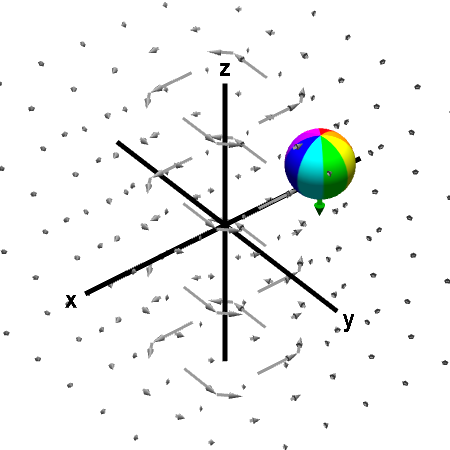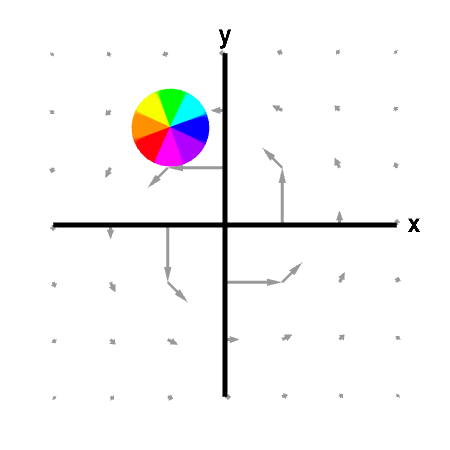# Math Insight

### Applet: Sphere rotating in opposite direction of macroscopic circulationWhen the center of the sphere is fixed, it rotates in a clockwise direction when viewed from the positive $z$-axis, corresponding to a downward pointing curl (green arrow). On the other hand, the counterclockwise macroscopic circulation of the vector field around the $z$-axis is evident from the graph. This example illustrates that one cannot infer curl from the macroscopic circulation of the vector field. Macroscopic and microscopic circulation can be very different. First panel shows the full vector field; second panel shows its projection in the $xy$-plane.

This vector field is $$\dlvf(x,y,z) = \frac{(-y,x,0)}{(x^2+y^2)^{3/2}}$$ for $(x,y) \ne (0,0)$. One can compute that away from the $z$-axis, $$\curl \dlvf(x,y,z) = \frac{(0,0,-1)}{(x^2+y^2)^{3/2}}.$$

The vector field and its curl blow up near the $z$-axis (the vector field arrows would get much longer if we plotted then closer to the $z$-axis). Hence, the sphere spins faster and the curl vector grows longer, the closer you move the sphere to the $z$-axis.